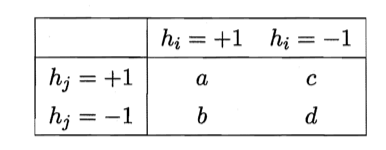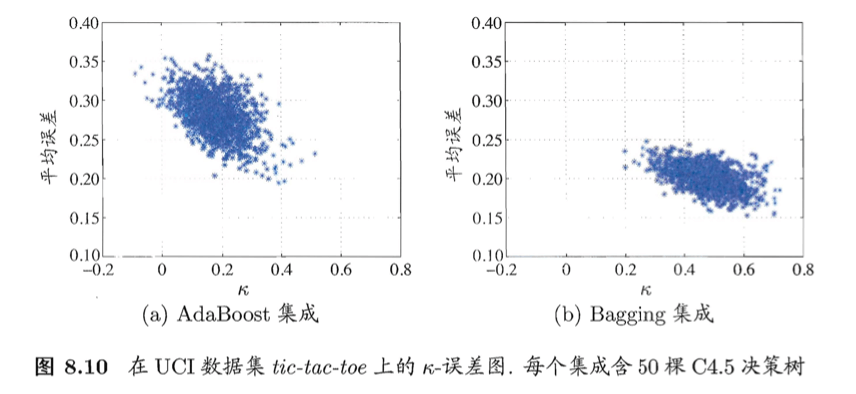# 【机器学习基础】第三十一课：集成学习之多样性

## 误差-分歧分解，多样性度量（不合度量、相关系数、Q-统计量、$\kappa$-统计量），多样性增强（数据样本扰动、输入属性扰动、输出表示扰动、算法参数扰动）

Posted by x-jeff on December 8, 2021

【机器学习基础】系列博客为参考周志华老师的《机器学习》一书，自己所做的读书笔记。

# 1.误差-分歧分解

【机器学习基础】第二十七课：集成学习之个体与集成一文中，欲构建泛化能力强的集成，个体学习器应“好而不同”。现在我们来做一个简单的理论分析。

$A(h_i \mid \mathbf{x})= (h_i(\mathbf{x})-H(\mathbf{x}))^2 \tag{1}$

$H(\mathbf{x})=\sum^T_{i=1} w_i h_i (\mathbf{x}) \tag{2}$

\begin{align} \bar{A}(h\mid \mathbf{x}) &= \sum^T_{i=1} w_i A(h_i \mid \mathbf{x}) \\&= \sum^T_{i=1} w_i (h_i(\mathbf{x})-H(\mathbf{x}))^2 \end{align} \tag{3}

$E(h_i \mid \mathbf{x})=(f(\mathbf{x})-h_i(\mathbf{x}))^2 \tag{4}$ $E(H \mid \mathbf{x})=(f(\mathbf{x})-H(\mathbf{x}))^2 \tag{5}$

\begin{align} \bar{A}(h\mid \mathbf{x}) &= \sum^T_{i=1} w_i (h_i(\mathbf{x})-H(\mathbf{x}))^2 \\&= \sum^T_{i=1} w_i (h_i(\mathbf{x})^2 -2h_i(\mathbf{x})H(\mathbf{x}) + H(\mathbf{x})^2) \\&= \sum^T_{i=1} w_i h_i(\mathbf{x})^2 -2\sum^T_{i=1} w_i h_i(\mathbf{x})H(\mathbf{x}) + \sum^T_{i=1} w_i H(\mathbf{x})^2 \\&= \sum^T_{i=1} w_i h_i(\mathbf{x})^2 -2 H(\mathbf{x}) \sum^T_{i=1} w_i h_i(\mathbf{x}) + H(\mathbf{x})^2 \sum^T_{i=1} w_i \\&= \sum^T_{i=1} w_i h_i(\mathbf{x})^2 -2 H(\mathbf{x}) \cdot H(\mathbf{x}) + H(\mathbf{x})^2 \\&= \sum^T_{i=1} w_i h_i(\mathbf{x})^2 -H(\mathbf{x})^2 \end{align} \tag{6}

$\sum^T_{i=1} w_i =1$。

\begin{align} \sum^T_{i=1} w_i E(h_i \mid \mathbf{x})-E(H\mid \mathbf{x}) &= \sum^T_{i=1} w_i (f(\mathbf{x})-h_i(\mathbf{x}))^2 - (f(\mathbf{x})-H(\mathbf{x}))^2 \\&= \sum^T_{i=1} w_i (f(\mathbf{x})^2 -2f(\mathbf{x})h_i(\mathbf{x}) + h_i(\mathbf{x})^2) - (f(\mathbf{x})^2 -2f(\mathbf{x})H(\mathbf{x}) + H(\mathbf{x})^2) \\&= f(\mathbf{x})^2 -2f(\mathbf{x}) \sum^T_{i=1} w_i h_i(\mathbf{x}) + \sum^T_{i=1} w_i h_i(\mathbf{x})^2 - f(\mathbf{x})^2 +2f(\mathbf{x})H(\mathbf{x}) - H(\mathbf{x})^2 \\&= f(\mathbf{x})^2 -2f(\mathbf{x})H(\mathbf{x}) + \sum^T_{i=1} w_i h_i(\mathbf{x})^2 - f(\mathbf{x})^2 +2f(\mathbf{x})H(\mathbf{x}) - H(\mathbf{x})^2 \\&= \sum^T_{i=1} w_i h_i(\mathbf{x})^2 - H(\mathbf{x})^2 \end{align} \tag{7}

\begin{align} \bar{A}(h\mid \mathbf{x}) &= \sum^T_{i=1} w_i E(h_i \mid \mathbf{x})-E(H\mid \mathbf{x}) \\&= \bar{E} (h\mid \mathbf{x})- E(H\mid \mathbf{x}) \end{align} \tag{8}

$\sum^T_{i=1} w_i \int A(h_i \mid \mathbf{x}) p(\mathbf{x}) d \mathbf{x}=\sum^T_{i=1} w_i \int E(h_i \mid \mathbf{x}) p(\mathbf{x}) d \mathbf{x} - \int E(H\mid \mathbf{x}) p(\mathbf{x}) d \mathbf{x} \tag{9}$

$E_i = \int E(h_i \mid \mathbf{x}) p(\mathbf{x}) d \mathbf{x} \tag{10}$ $A_i = \int A(h_i \mid \mathbf{x}) p(\mathbf{x}) d \mathbf{x} \tag{11}$

$E=\int E(H\mid \mathbf{x}) p(\mathbf{x}) d \mathbf{x} \tag{12}$

$E=\bar{E}-\bar{A} \tag{13}$

# 2.多样性度量👉不合度量（disagreement measure）：

$dis_{ij} = \frac{b+c}{m} \tag{14}$

$dis_{ij}$的值域为$[0,1]$。值越大则多样性越大。

👉相关系数（correlation coefficient）：

$\rho_{ij} = \frac{ad-bc}{\sqrt{(a+b)(a+c)(c+d)(b+d)}} \tag{15}$

$\rho_{ij}$的值域为$[-1,1]$。若$h_i$与$h_j$无关，则值为0；若$h_i$与$h_j$正相关则值为正，否则为负。

👉Q-统计量（Q-statistic）：

$Q_{ij}=\frac{ad-bc}{ad+bc} \tag{16}$

$Q_{ij}$与相关系数$\rho_{ij}$的符号相同，且$\lvert Q_{ij} \rvert \leqslant \lvert \rho_{ij} \rvert$。

👉$\kappa$-统计量（$\kappa$-statistic）：

$\kappa = \frac{p_1-p_2}{1-p_2} \tag{17}$

$p_1 = \frac{a+d}{m} \tag{18}$ $p_2 = \frac{(a+b)(a+c)+(c+d)(b+d)}{m^2} \tag{19}$# 3.多样性增强

## 3.4.算法参数扰动